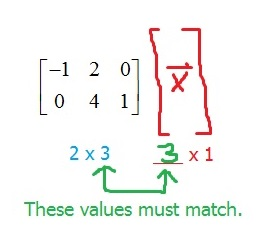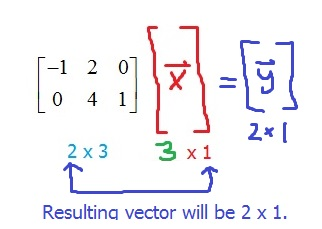# Matrix transformations

A matrix transformation is a transformation whose rule is based on multiplication of a vector by a matrix. This type of transformation is of particular interest to us in studying linear algebra as matrix transformations are always linear transformations. Further, we can use the matrix that defines the transformation to better understand other properties of the transformation itself.

Mathematically, a transformation T is a matrix transformation if we can write$T(\vec{x}) = A\vec{x}$ for some matrix A.

## Example of a matrix transformation

Let$T(\vec{x}) = A\vec{x}$ where$A = \begin{bmatrix} -1 & 2 & 0\\ 0 & 4 & 1\\ \end{bmatrix}$.

### Observations

The example above shows a matrix transformation, since T is defined through multiplying the matrix A and the input vector$\vec{x}$. To better understand this transformation, we will make a few observations.

### The domain of this transformation is$\mathbb{R}^3$

If we “plug in” a vector$\vec{x}$, we find its image through multiplying the vector by A. But, this multiplication is not always defined! This will only be defined if the number of entries in$\vec{x}$ matches the number of columns in A. This means that when we write out the size of the matrix and the size of the vector, the “inner numbers” must match.### The codomain of this transformation is$\mathbb{R}^2$

When multiplying a matrix and a vector, the result is determined by the “outer numbers” in our diagram above.What is happening mathematically to make this true?

If we let$\vec{x} = \begin{bmatrix} x_1 \\ x_2 \\ x_3 \\ \end{bmatrix}$, then:$T(\vec{x}) = A\vec{x} = \begin{bmatrix} -1 & 2 & 0\\ 0 & 4 & 1\\ \end{bmatrix} \begin{bmatrix} x_1 \\ x_2 \\ x_3 \\ \end{bmatrix} = x_1 \begin{bmatrix} -1 \\ 0\\ \end{bmatrix} + x_2\begin{bmatrix} 2 \\ 4\\ \end{bmatrix} + x_3\begin{bmatrix} 0 \\ 1\\ \end{bmatrix}$

In other words, the image of$\vec{x}$ will be a linear combination of the columns of A. Since the columns each have 2 entries, we know that the columns are vectors in$\mathbb{R}^2$ and so any linear combination will also be in$\mathbb{R}^2$. Vectors in$\mathbb{R}^2$ have dimension 2 x 1.

From these two observations, we know that:$T: \mathbb{R}^3 \rightarrow \mathbb{R}^2$

That is, T maps vectors in$\mathbb{R}^3$ to vectors in$\mathbb{R}^2$.

### To find the image of any vector, we just need to multiply

Suppose that we wanted to find the image of the vector$\begin{bmatrix} 6 \\ 1 \\ 3 \\ \end{bmatrix}$ under T. Then, we would simply “plug” this vector into T.$T\left(\begin{bmatrix} 6 \\ 1 \\ 3 \\ \end{bmatrix}\right) = \begin{bmatrix} -1 & 2 & 0\\ 0 & 4 & 1\\ \end{bmatrix} \begin{bmatrix} 6 \\ 1 \\ 3 \\ \end{bmatrix} = 6 \begin{bmatrix} -1 \\ 0\\ \end{bmatrix} + 1\begin{bmatrix} 2 \\ 4\\ \end{bmatrix} + 3\begin{bmatrix} 0 \\ 1\\ \end{bmatrix} = \begin{bmatrix} -4 \\ 7 \\ \end{bmatrix}$

Since$T\left(\begin{bmatrix} 6 \\ 1 \\ 3 \\ \end{bmatrix}\right) = \begin{bmatrix} -4 \\ 7 \\ \end{bmatrix}$, the image of$\begin{bmatrix} 6 \\ 1 \\ 3 \\ \end{bmatrix}$ under T is$\begin{bmatrix} -4 \\ 7 \\ \end{bmatrix}$.

### T must be linear

Every matrix transformation is a linear transformation. You can review a proof of this idea here: Proof that every matrix transformation is a linear transformation